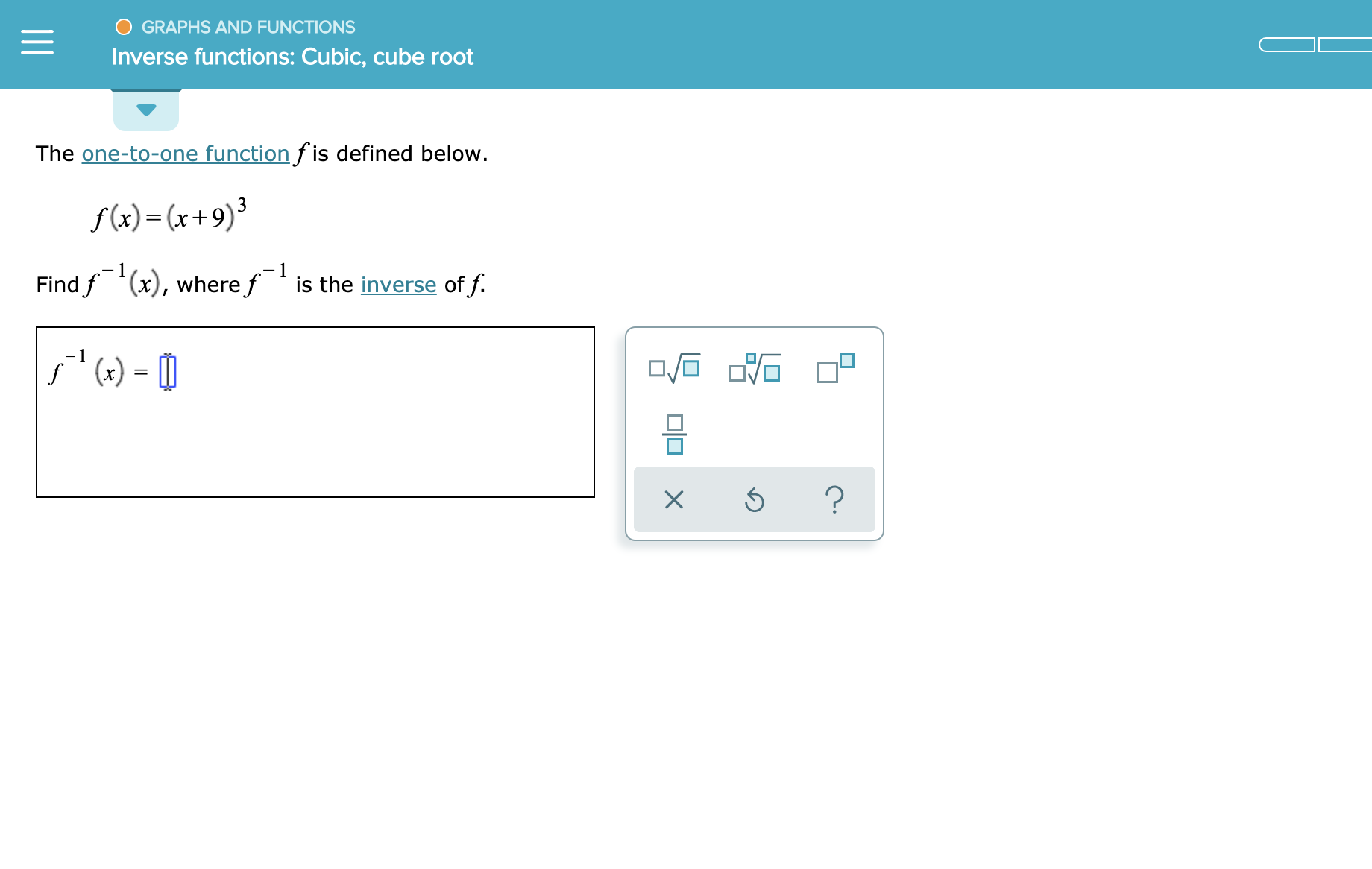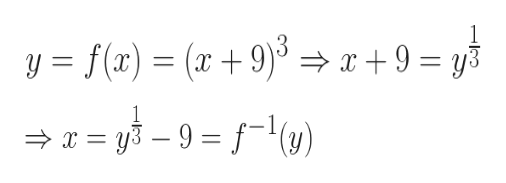GRAPHS AND FUNCTIONSInverse functions: Cubic, cube rootThe one-to-one function fis defined below.f()(x+9)-1Find f (x), wheref is the inverse of f.'()?미미 x

Question

see attachmenthelp_outlineImage TranscriptioncloseGRAPHS AND FUNCTIONS Inverse functions: Cubic, cube root The one-to-one function fis defined below. f()(x+9) -1 Find f (x), wheref is the inverse of f. '() ? 미미 x fullscreen
Step 1

We will follow the following two steps to figure out the inverse function:

1. Assume, y = f(x). Hence, x = f-1(y). We will therefore express x as a function of y. That will be f-1(y)

2. In the expression for f-1(y), we will then replace y by x to get f-1(x)

Step 2

Please see the wh...help_outlineImage Transcriptionclose1 yf(x) = (x +9)x+9 = y X 1 1 fullscreen

Want to see the full answer?

See Solution

Want to see this answer and more?

Our solutions are written by experts, many with advanced degrees, and available 24/7

See Solution
Tagged in

Calculus